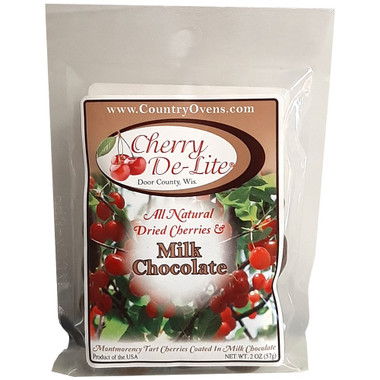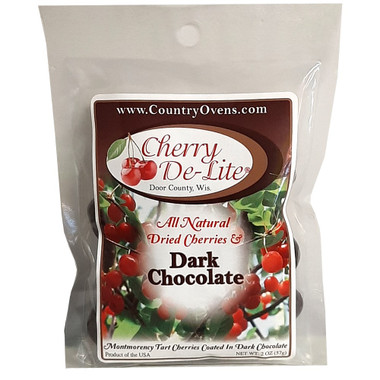# Dried Cherries

Cherries Dried to Make The Perfect Snack!

Showing 1 - of 8

•Quantity Decrease Quantity Increase Quantity
•Quantity Decrease Quantity Increase Quantity
•Quantity Decrease Quantity Increase Quantity
•Quantity Decrease Quantity Increase Quantity
•Quantity Decrease Quantity Increase Quantity
•Quantity Decrease Quantity Increase Quantity
•Quantity Decrease Quantity Increase Quantity
•Quantity Decrease Quantity Increase Quantity